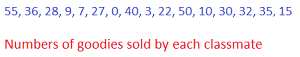# Box & Whisker Plot: Lesson for Kids

I have a Bachelor's degree in Elementary Education and Spanish. I have taught for 5 years in bilingual classrooms of various elementary grade levels.

What do you do when you have a lot of numbers that are spread out, and you want to group them into sets and graph them? In this lesson, you will learn how to create and read a box and whisker plot which is perfect to use with that type of data.

## Uses of a Box and Whisker Plot

Cookies, cupcakes, fruit pies! Oh my! Your school just had its annual bake sale, and each class had to make and sell their sweets. Your teacher wants to see how well the 16 students in her class did and suggests using a box and whisker plot, but what exactly is that?

A box and whisker plot is a special type of graph that is used to show groups of number data and how they are spread. It shows the median, which is the middle value of the numbers in your data, the lowest number, the highest number and the quartiles, which divides the data into four equal groups. Quartiles are like quarters. There are 4 quarters in a dollar, each of which is worth 25 cents, so there are 4 quartiles in a box and whisker plot, each showing 25% of the number data. Let's take a look at our bake sale numbers and put this information to use.

## Creating a Box and Whisker PlotTake a look at the image which shows the numbers of goodies each classmate sold. Now it's time to create your own box and whisker plot! Your teacher tells you to:

1. Order the numbers from least to greatest:

• 0, 3, 7, 9, 10, 15, 22, 27, 28, 30, 32, 35, 36, 40, 50, 55

2. Find the median and record it on the line. If there is an odd amount of number data, this will be the middle number. If there is an even amount of number data, take the average of the two numbers in the middle. This will create halves.

• We have 16 numbers, so our two middle numbers are 27 and 28. Add them together and divide by two to find the average = 27.5. This is our median.

3. Plot the lowest number at the beginning and the highest number at the end of the number line.

• 0 is our lower extreme and 55 is our upper extreme

4. Find the median for each half of the data - this is dividing the data into quartiles. To do this, you must repeat the process for finding the median, but do it for each half. Record both numbers on the line.

• Our first half of the data are the first eight numbers. The two middle numbers are 9 and 10. So, the average is 9.5. Our second half of data are the last eight numbers. The middle numbers are 35 and 36, so the average is 35.5.

To unlock this lesson you must be a Study.com Member.

### Register to view this lesson

Are you a student or a teacher?

#### See for yourself why 30 million people use Study.com

##### Become a Study.com member and start learning now.
Back
What teachers are saying about Study.com

### Earning College Credit

Did you know… We have over 200 college courses that prepare you to earn credit by exam that is accepted by over 1,500 colleges and universities. You can test out of the first two years of college and save thousands off your degree. Anyone can earn credit-by-exam regardless of age or education level.